# Package Modelica.​Mechanics.​MultiBody.​Examples.​ElementaryElementary examples to demonstrate various features of the MultiBody library

### Information

This package contains elementary example models to demonstrate the usage of the MultiBody library

#### Content

ModelDescription
DoublePendulum
DoublePendulumInitTip
Simple double pendulum with two revolute joints and two bodies. In DoublePendulumInitTip, the initial position of the pendulum tip is given instead of common initialization by pendulum angles.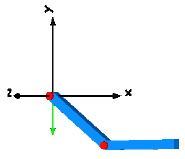ForceAndTorque Demonstrates usage of Forces.ForceAndTorque element.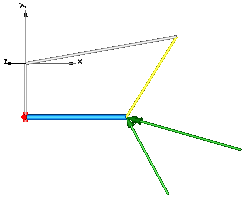FreeBody Free flying body attached by two springs to environment.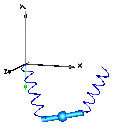InitSpringConstant Determine spring constant such that system is in steady state at given position.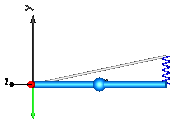LineForceWithTwoMasses Demonstrates a line force with two point masses using a Joints.Assemblies.JointUPS and alternatively a Forces.LineForceWithTwoMasses component.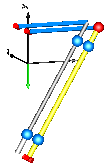Pendulum Simple pendulum with one revolute joint and one body.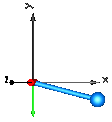PendulumWithSpringDamper Simple spring/damper/mass system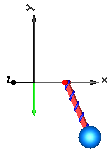PointGravity Two bodies in a point gravity field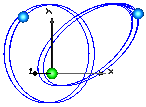PointGravityWithPointMasses Two point masses in a point gravity field (rotation of bodies is neglected)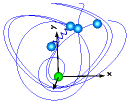PointGravityWithPointMasses2 Rigidly connected point masses in a point gravity field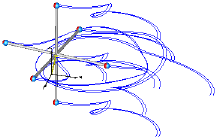RollingWheel Single wheel rolling on ground starting from an initial speed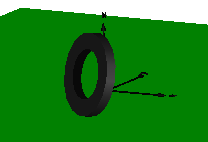RollingWheelSetDriving Rolling wheel set that is driven by torques driving the wheels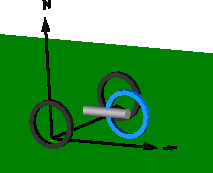RollingWheelSetPulling Rolling wheel set that is pulled by a force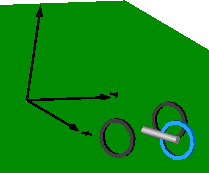SpringDamperSystem Spring/damper system with a prismatic joint and attached on free flying body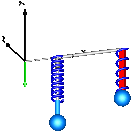SpringMassSystem Mass attached via a prismatic joint and a spring to the world frame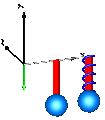SpringWithMass Point mass hanging on a spring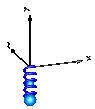ThreeSprings 3-dimensional springs in series and parallel connection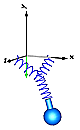HeatLosses Demonstrate the modeling of heat losses.
UserDefinedGravityField Demonstrate the modeling of a user-defined gravity field.
Surfaces Demonstrate the visualization of a sine surface, as well as a torus and a wheel constructed from a surface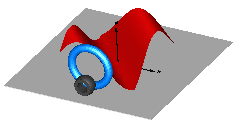Extends from `Modelica.​Icons.​ExamplesPackage` (Icon for packages containing runnable examples).

### Package Contents

NameDescription
`DoublePendulum`Simple double pendulum with two revolute joints and two bodies
`DoublePendulumInitTip`Demonstrate how to initialize a double pendulum so that its tip starts at a predefined position
`ForceAndTorque`Demonstrate usage of ForceAndTorque element
`FreeBody`Free flying body attached by two springs to environment
`HeatLosses`Demonstrate the modeling of heat losses
`InitSpringConstant`Determine spring constant such that system is in steady state at given position
`LineForceWithTwoMasses`Demonstrate line force with two point masses using a JointUPS and alternatively a LineForceWithTwoMasses component
`Pendulum`Simple pendulum with one revolute joint and one body
`PendulumWithSpringDamper`Simple spring/damper/mass system
`PointGravity`Two point masses in a point gravity field
`PointGravityWithPointMasses`Two point masses in a point gravity field (rotation of bodies is neglected)
`PointGravityWithPointMasses2`Rigidly connected point masses in a point gravity field
`RollingWheel`Single wheel rolling on ground starting from an initial speed
`RollingWheelSetDriving`Rolling wheel set that is driven by torques driving the wheels
`RollingWheelSetPulling`Rolling wheel set that is pulled by a force
`SpringDamperSystem`Simple spring/damper/mass system
`SpringMassSystem`Mass attached with a spring to the world frame
`SpringWithMass`Point mass hanging on a spring
`Surfaces`Demonstrate the visualization of a sine surface, as well as a torus and a wheel constructed from a surface
`ThreeSprings`3-dim. springs in series and parallel connection
`UserDefinedGravityField`Demonstrate the modeling of a user-defined gravity field
`Utilities`Utility classes used by elementary multi-body example models

## Model Modelica.​Mechanics.​MultiBody.​Examples.​Elementary.​DoublePendulumSimple double pendulum with two revolute joints and two bodies

### Information

This example demonstrates that by using joint and body elements animation is automatically available. Also the revolute joints are animated. Note, that animation of every component can be switched of by setting the first parameter animation to false or by setting enableAnimation in the world object to false to switch off animation of all components.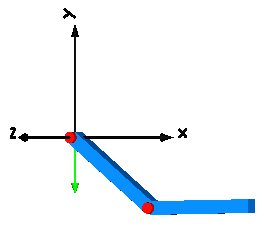Extends from `Modelica.​Icons.​Example` (Icon for runnable examples).

## Model Modelica.​Mechanics.​MultiBody.​Examples.​Elementary.​DoublePendulumInitTipDemonstrate how to initialize a double pendulum so that its tip starts at a predefined position

### Information

This example demonstrates at hand of a double pendulum, how non-standard initialization can be defined: The absolute position of the pendulum tip, and its absolute speed shall be initially defined. This can be performed with the Joints.FreeMotionScalarInit joint that allows to initialize individual elements of its relative vectors. In this case, the x-, and y-coordinates of the relative position vector (visualized by the yellow arrow in the figure below) and of its derivative shall have a defined value at initial time. The configuration of the double pendulum at the initial time is shown below, where the tip position is required to have the coordinates x=0.7, y=0.3.

Setting only the tip's start position results in an ambiguous initialization since two valid solutions for `revolute1.phi` and `revolute2.phi` exist. Moreover, the calculated angles `revolute1.phi` and `revolute2.phi` can be a multiple of expected solution `phi1` and `phi2`,

• revolute1.phi(k1) = phi1 + 2 π k1,
• revolute2.phi(k2) = phi2 + 2 π k2.

To clearly indicate the preferred solution, guess initial angles can be additionally given. In this example, it is simply done by `revolute2.phi.start = Modelica.Constants.pi/2`.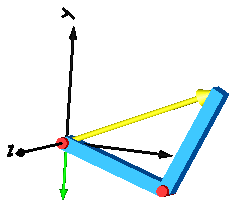Extends from `Modelica.​Icons.​Example` (Icon for runnable examples).

## Model Modelica.​Mechanics.​MultiBody.​Examples.​Elementary.​ForceAndTorqueDemonstrate usage of ForceAndTorque element

### Information

In this example the usage of the general force element "ForceAndTorque" is shown. A "ForceAndTorque" element is connected between a body and a fixed point in the world system. The force and torque is defined by the "Constant" block. The two vectors are resolved in the coordinate system defined by the "fixedRotation" component that is fixed in the world system:

The animation view at time = 0 is shown in the figure below. The yellow line is directed from frame_a to frame_b of the forceAndTorque component. The green arrow characterizes the force acting at the body whereas the green double arrow characterizes the torque acting at the body. The lengths of the two vectors are proportional to the lengths of the force and torque vectors (constant scaling factors are defined as parameters in the forceAndTorque component):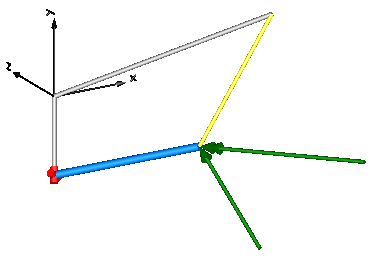Extends from `Modelica.​Icons.​Example` (Icon for runnable examples).

## Model Modelica.​Mechanics.​MultiBody.​Examples.​Elementary.​FreeBodyFree flying body attached by two springs to environment

### Information

This example demonstrates:

• The animation of spring and damper components
• A body can be freely moving without any connection to a joint. In this case body coordinates are used automatically as states (whenever joints are present, it is first tried to use the generalized coordinates of the joints as states).
• If a body is freely moving, the initial position and velocity of the body can be defined with the "Initialization" menu as shown with the body "body1" in the left part (click on "Initialization").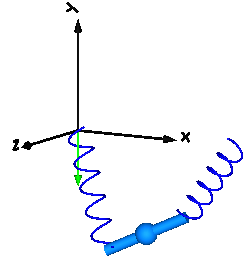Extends from `Modelica.​Icons.​Example` (Icon for runnable examples).

### Parameters

TypeNameDefaultDescription
`Boolean``animation``true`= true, if animation shall be enabled

## Model Modelica.​Mechanics.​MultiBody.​Examples.​Elementary.​InitSpringConstantDetermine spring constant such that system is in steady state at given position

### Information

This example demonstrates a non-standard type of initialization by calculating a spring constant such that a simple pendulum is at a defined position in steady state.

The goal is that the pendulum should be in steady state when the rotation angle of the pendulum is zero. The spring constant of the spring shall be calculated during initialization such that this goal is reached.

The pendulum has one degree of freedom, i.e., two states. Therefore, two additional equations have to be provided for initialization. However, parameter "c" of the spring component is defined with attribute "fixed = false", i.e., the value of this parameter is computed during initialization. Therefore, there is one additional equation required during initialization. The 3 initial equations are the rotational angle of the revolute joint and its first and second derivative. The latter ones are zero, in order to initialize in steady state. By setting the start values of phi, w, a to zero and their fixed attributes to true, the required 3 initial equations are defined.

After translation, this model is initialized in steady-state. The spring constant is computed as c = 49.05 N/m. An animation of this simulation is shown in the figure below.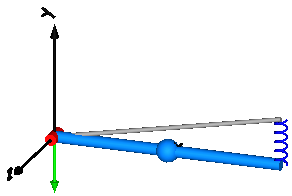Extends from `Modelica.​Icons.​Example` (Icon for runnable examples).

## Model Modelica.​Mechanics.​MultiBody.​Examples.​Elementary.​LineForceWithTwoMassesDemonstrate line force with two point masses using a JointUPS and alternatively a LineForceWithTwoMasses component

### Information

It is demonstrated how to implement line force components that shall have mass properties. Two alternative implementations are given:

• With JointUPS:
Modelica.Mechanics.MultiBody.Joints.Assemblies.JointUPS is an aggregation of a universal, a prismatic and a spherical joint that approximates a real force component, such as a hydraulic cylinder. At the two frames of the prismatic joint (frame_ia, frame_ib of jointUPS) two bodies are attached. The parameters are selected such that the center of masses of the two bodies are located on the line connecting frame_a and frame_b of the jointUPS component. Both bodies have the same mass and the inertia tensor is set to zero, i.e., the two bodies are treated as point masses.
• With LineForceWithTwoMasses:
Modelica.Mechanics.MultiBody.Forces.LineForceWithTwoMasses is a line force component with the built-in property that two point masses are located on the line on which the line force is acting. The parameters are selected in such a way that the same system as with the jointUPS component is described.

In both cases, a linear 1-dimensional translational damper from the Modelica.Mechanics.Translational library is used as line force between the two attachment points. Simulate this system and plot the differences of the cut forces at both sides of the line force component ("rod_f_diff" and "body_f_diff"). Both vectors should be zero (depending on the chosen relative tolerance of the integration, the difference is in the order of 1e-10 ... 1e-15).

Note, that the implementation with the LineForceWithTwoMasses component is simpler and more convenient. An animation of this simulation is shown in the figure below. The system on the left side in the front is the animation with the LineForceWithTwoMasses component whereas the system on the right side in the back is the animation with the JointUPS component.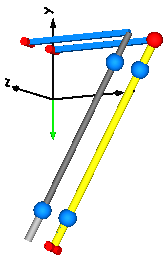Extends from `Modelica.​Icons.​Example` (Icon for runnable examples).

### Parameters

TypeNameDefaultDescription
`Mass``m``1`Mass of point masses

## Model Modelica.​Mechanics.​MultiBody.​Examples.​Elementary.​PendulumSimple pendulum with one revolute joint and one body

### Information

This simple model demonstrates that by just dragging components default animation is defined that shows the structure of the assembled system.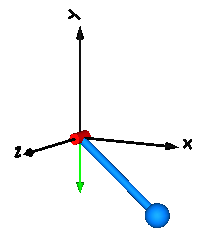Extends from `Modelica.​Icons.​Example` (Icon for runnable examples).

## Model Modelica.​Mechanics.​MultiBody.​Examples.​Elementary.​PendulumWithSpringDamperSimple spring/damper/mass system

### Information

A body is attached on a revolute and prismatic joint. A 3-dim. spring and a 3-dim. damper are connected between the body and a point fixed in the world frame: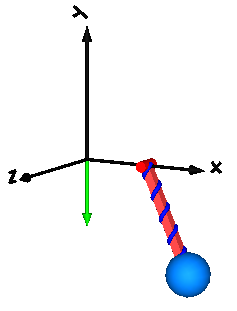Extends from `Modelica.​Icons.​Example` (Icon for runnable examples).

### Parameters

TypeNameDefaultDescription
`Boolean``animation``true`= true, if animation shall be enabled

## Model Modelica.​Mechanics.​MultiBody.​Examples.​Elementary.​PointGravityTwo point masses in a point gravity field

### Information

This model demonstrates a point gravity field. Two bodies are placed in the gravity field. The initial positions and velocities of these bodies are selected such that one body rotates on a circle and the other body rotates on an ellipse around the center of the point gravity field.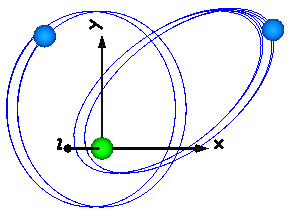Extends from `Modelica.​Icons.​Example` (Icon for runnable examples).

## Model Modelica.​Mechanics.​MultiBody.​Examples.​Elementary.​PointGravityWithPointMassesTwo point masses in a point gravity field (rotation of bodies is neglected)

### Information

This model demonstrates the usage of model Parts.PointMass in a point gravity field. The PointMass model has the feature that the rotation is not taken into account and can therefore also not be calculated. This example demonstrates two cases where this does not matter: If a PointMass is not connected (body1, body2), the orientation object in these point masses is set to a unit rotation. If a PointMass is connected by a line force element, such as the used Forces.LineForceWithMass component, then the orientation object is set to a unit rotation within the line force element. These are the two cases where the rotation is automatically set to a default value, when the physical system does not provide the equations.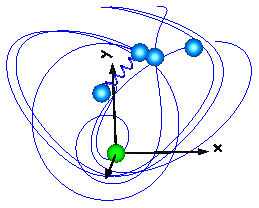Extends from `Modelica.​Icons.​Example` (Icon for runnable examples).

## Model Modelica.​Mechanics.​MultiBody.​Examples.​Elementary.​PointGravityWithPointMasses2Rigidly connected point masses in a point gravity field

### Information

This model demonstrates the usage of model Parts.PointMass in a point gravity field. 6 point masses are connected rigidly together. Translating such a model results in an error, because point masses do not define an orientation object. The example demonstrates that in such a case (when the orientation object is not defined by an object that is connected to a point mass), a "MultiBody.Joints.FreeMotion" joint has to be used, to define the degrees of freedom of this structure.

In order to demonstrate that this approach is correct, in model "referenceSystem", the same system is again provided, but this time modeled with a generic body (Parts.Body) where the inertia tensor is set to zero. In this case, no FreeMotion object is needed because every body provides its absolute translational and rotational position and velocity as potential states.

The two systems should move exactly in the same way. The system with the PointMasses object visualizes the point masses in "red", whereas the "referenceSystem" shows its bodies in "blue".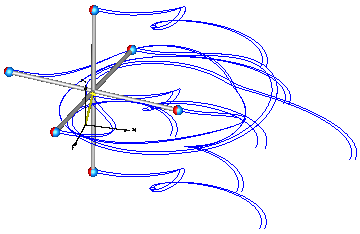Extends from `Modelica.​Icons.​Example` (Icon for runnable examples).

## Model Modelica.​Mechanics.​MultiBody.​Examples.​Elementary.​SpringDamperSystemSimple spring/damper/mass system

### Information

This example demonstrates:

• The animation of spring and damper components
• A body can be freely moving without any connection to a joint. In this case body coordinates are used automatically as states (whenever joints are present, it is first tried to use the generalized coordinates of the joints as states).
• If a body is freely moving, the initial position and velocity of the body can be defined with the "Initialization" menu as shown with the body "body1" in the left part (click on "Initialization").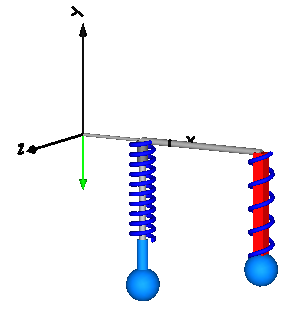Extends from `Modelica.​Icons.​Example` (Icon for runnable examples).

### Parameters

TypeNameDefaultDescription
`Boolean``animation``true`= true, if animation shall be enabled

## Model Modelica.​Mechanics.​MultiBody.​Examples.​Elementary.​SpringMassSystemMass attached with a spring to the world frame

### Information

This example shows the two different ways how force laws can be utilized:

• In the left system a body is attached via a prismatic joint to the world frame. The prismatic joint has two 1-dimensional translational flanges (called "support" and "axis") that allows to connect elements from the Modelica.Mechanics.Translational library between the support and the axis connector. The effect is that the force generated by the 1-dimensional elements acts as driving force in the axis of the prismatic joint. In the example a simple spring is used.
The advantage of this approach is that the many elements from the Translational library can be easily used here and that this implementation is usually more efficient as when using 3-dimensional springs.
• In the right system the same model is defined. The difference is that a 3-dimensional spring from the Modelica.Mechanics.MultiBody.Forces library is used. This has the advantage to get a nice animation of the force component.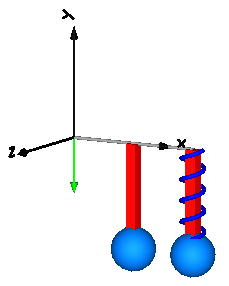Extends from `Modelica.​Icons.​Example` (Icon for runnable examples).

### Parameters

TypeNameDefaultDescription
`Boolean``animation``true`= true, if animation shall be enabled

## Model Modelica.​Mechanics.​MultiBody.​Examples.​Elementary.​SpringWithMassPoint mass hanging on a spring

### Information

This example shows that a force component may have a mass. The 3-dimensional spring as used in this example, has an optional point mass between the two points where the spring is attached. In the animation, this point mass is represented by a small, light blue, sphere.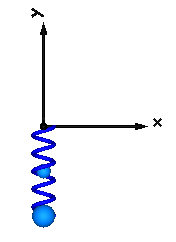Extends from `Modelica.​Icons.​Example` (Icon for runnable examples).

## Model Modelica.​Mechanics.​MultiBody.​Examples.​Elementary.​ThreeSprings3-dim. springs in series and parallel connection

### Information

This example demonstrates that 3-dimensional line force elements (here: Modelica.Mechanics.MultiBody.Forces.Spring elements) can be connected together in series without having a body with mass at the connection point (as usually required by multi-body programs). This is advantageous since stiff systems can be avoided, say, due to a stiff spring and a small mass at the connection point.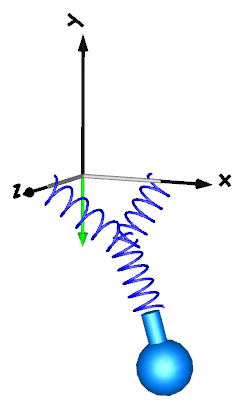For a more thorough explanation, see MultiBody.UsersGuide.Tutorial.ConnectionOfLineForces.

Extends from `Modelica.​Icons.​Example` (Icon for runnable examples).

### Parameters

TypeNameDefaultDescription
`Boolean``animation``true`= true, if animation shall be enabled

## Model Modelica.​Mechanics.​MultiBody.​Examples.​Elementary.​RollingWheelSingle wheel rolling on ground starting from an initial speed

### Information

Demonstrates how a single wheel is rolling on ground (starting with an initial velocity).

Extends from `Modelica.​Icons.​Example` (Icon for runnable examples).

## Model Modelica.​Mechanics.​MultiBody.​Examples.​Elementary.​RollingWheelSetDrivingRolling wheel set that is driven by torques driving the wheels

### Information

Demonstrates how a RollingWheelSet (two wheels rigidly coupled together) is rolling on ground when driven by some external torques.

Extends from `Modelica.​Icons.​Example` (Icon for runnable examples).

## Model Modelica.​Mechanics.​MultiBody.​Examples.​Elementary.​RollingWheelSetPullingRolling wheel set that is pulled by a force

### Information

Demonstrates how a RollingWheelSet (two wheels rigidly coupled together) is rolling on ground when pulled by an external force..

Extends from `Modelica.​Icons.​Example` (Icon for runnable examples).

## Model Modelica.​Mechanics.​MultiBody.​Examples.​Elementary.​HeatLossesDemonstrate the modeling of heat losses

### Information

This model demonstrates how to model the dissipated power of a multi-body force element by enabling the heatPort of all components and connecting these heatPorts via a convection element to the environment. The total heat flow generated by the elements of this multi-body system and transported to the environment is present in variable convection.fluid.

Extends from `Modelica.​Icons.​Example` (Icon for runnable examples).

## Model Modelica.​Mechanics.​MultiBody.​Examples.​Elementary.​UserDefinedGravityFieldDemonstrate the modeling of a user-defined gravity field

### Information

This example demonstrates a user defined gravity field. Function "world.gravityAcceleration" is redeclared to function theoreticalNormalGravityWGS84 that computes the theoretical gravity of the WGS84 ellipsoid earth model at and close to the earth ellipsoid surface. In the gravity field, a large, single pendulum is present. Via parameter "geodeticLatitude", the geodetic latitude on the earth can be defined, where the pendulum is present. The world frame is located at the WGS84 earth ellipsoid at this latitude. The result variable "gravity" is the gravity vector at the center of mass of the pendulum mass. Since the height of this mass is changing, the value of the gravity is also changing (the difference is in the order of 0.00001).

The result of the simulation is slightly different at the equator (geodeticLatitude=0) and at the poles (geodeticLatitude=90). For example, after 10 s of simulation time the rotation angle of the pendulum, rev.phi, has the following values:

Extends from `Modelica.​Icons.​Example` (Icon for runnable examples).

### Parameters

TypeNameDefaultDescription
`Angle_deg``geodeticLatitude``0`Geodetic latitude
`Position``height``20`Height of pendulum attachment point over WGS84 earth ellipsoid

## Model Modelica.​Mechanics.​MultiBody.​Examples.​Elementary.​SurfacesDemonstrate the visualization of a sine surface, as well as a torus and a wheel constructed from a surface

### Information

This example demonstrates the use of the Surface visualizer that visualizes a moving, parameterized surface. The "sine-wave" surface is a direct application of the surface model. Furthermore, the "torus" surface is an instance of Torus, the "wheel" surface is an instance of VoluminousWheel, and the "pipeWithScalarField surface is an instance of PipeWithScalarField. All latter visual shapes are constructed with the surface model. The following image shows a screen-shot of this example model: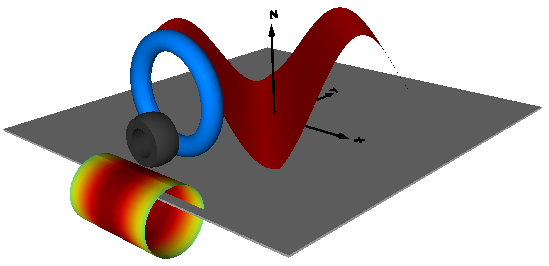Extends from `Modelica.​Icons.​Example` (Icon for runnable examples).

### Parameters

TypeNameDefaultDescription
`Real``x_min``-1`Minimum value of x
`Real``x_max``1`Maximum value of x
`Real``y_min``-1`Minimum value of y
`Real``y_max``1`Maximum value of y
`Real``z_min``0`Minimum value of z
`Real``z_max``1`Maximum value of z

Generated 2018-12-12 12:11:33 EST by MapleSim.